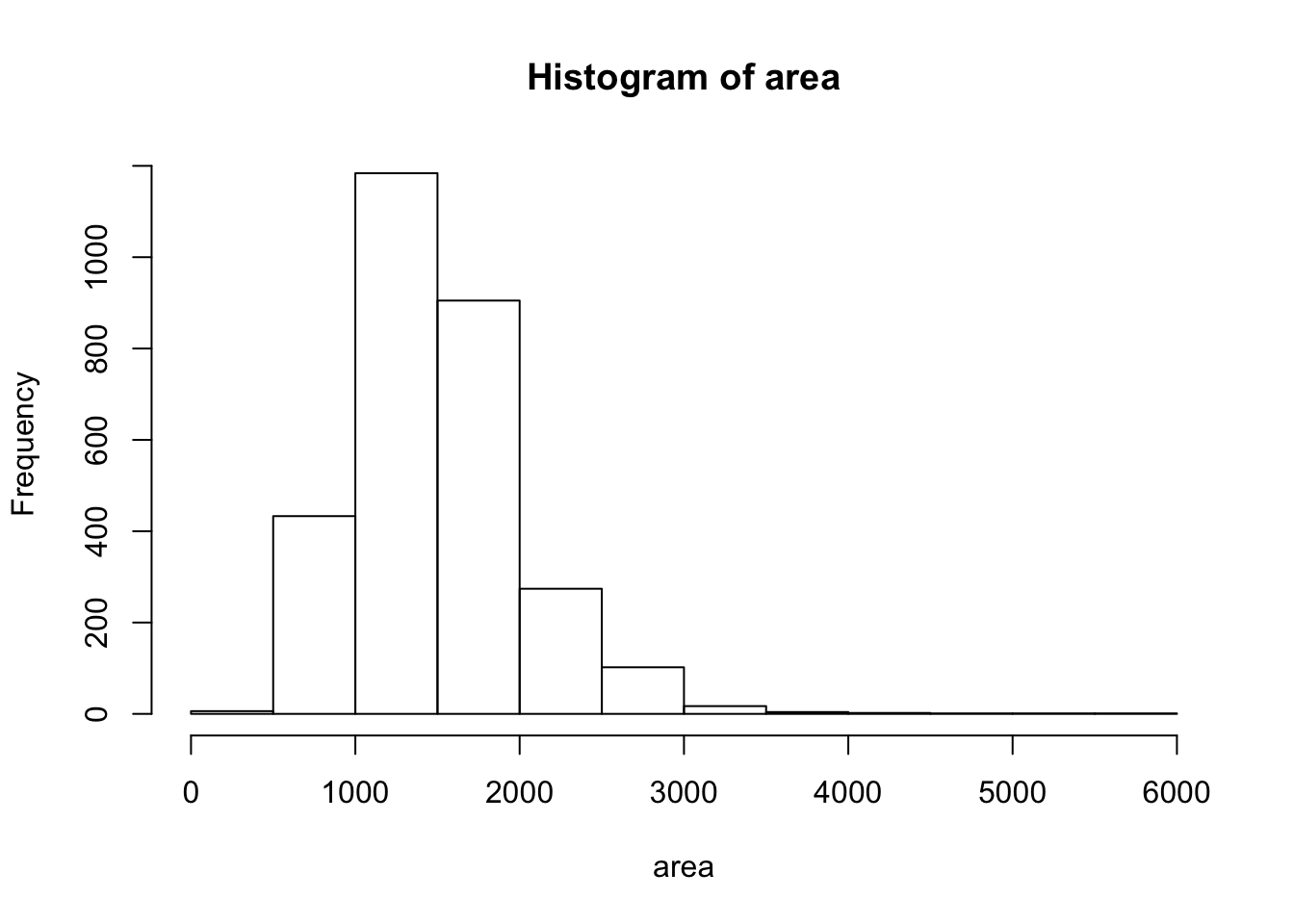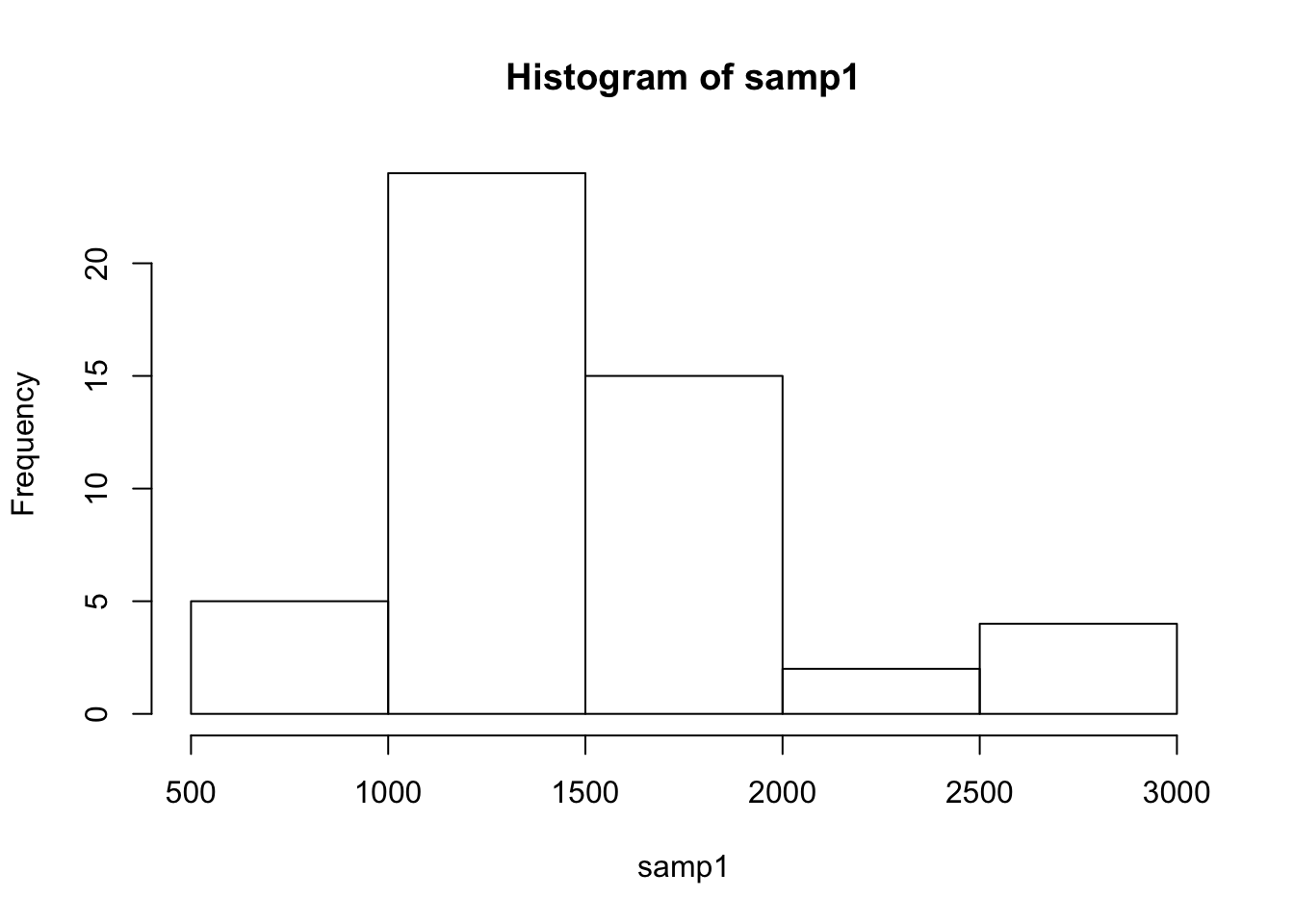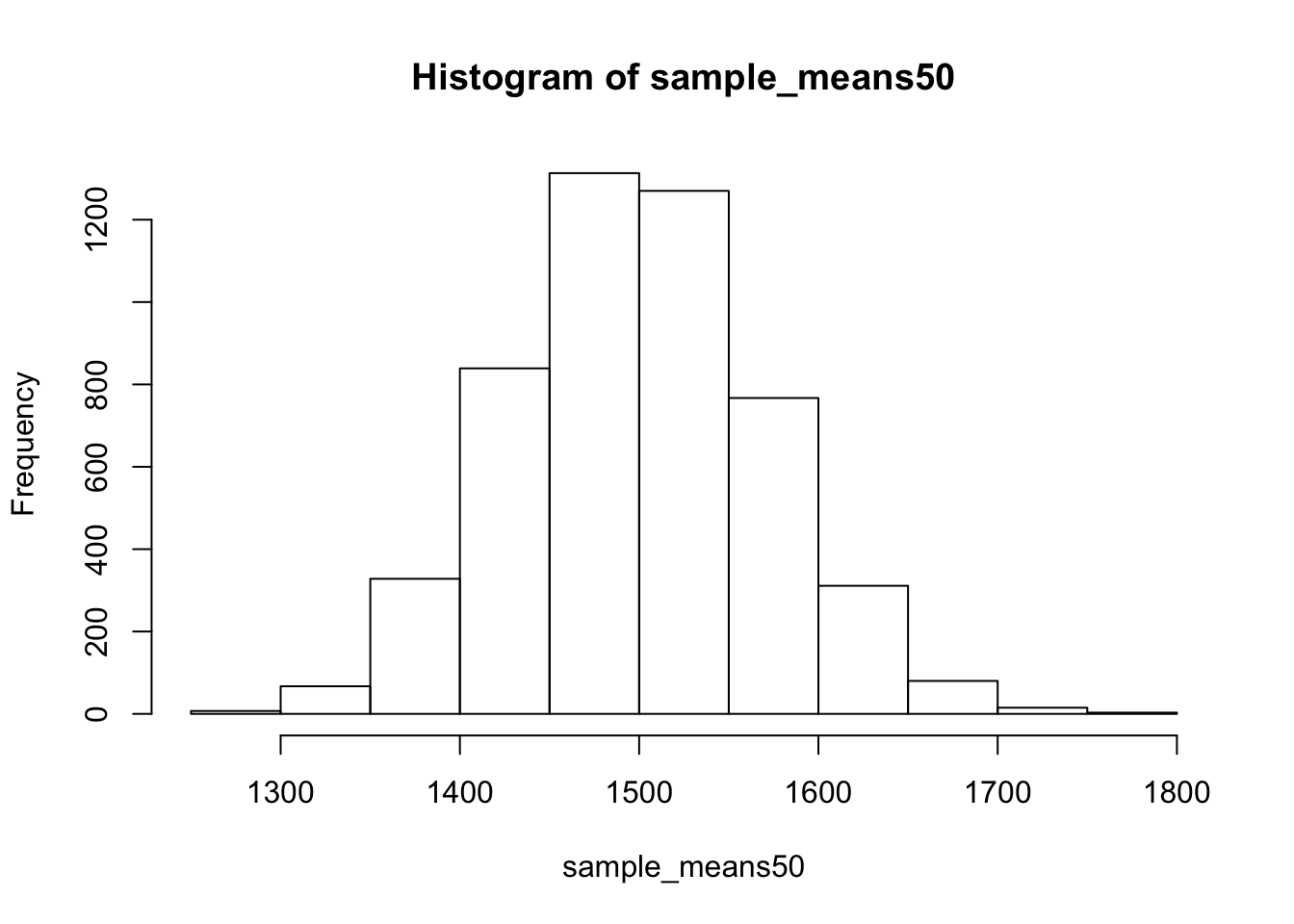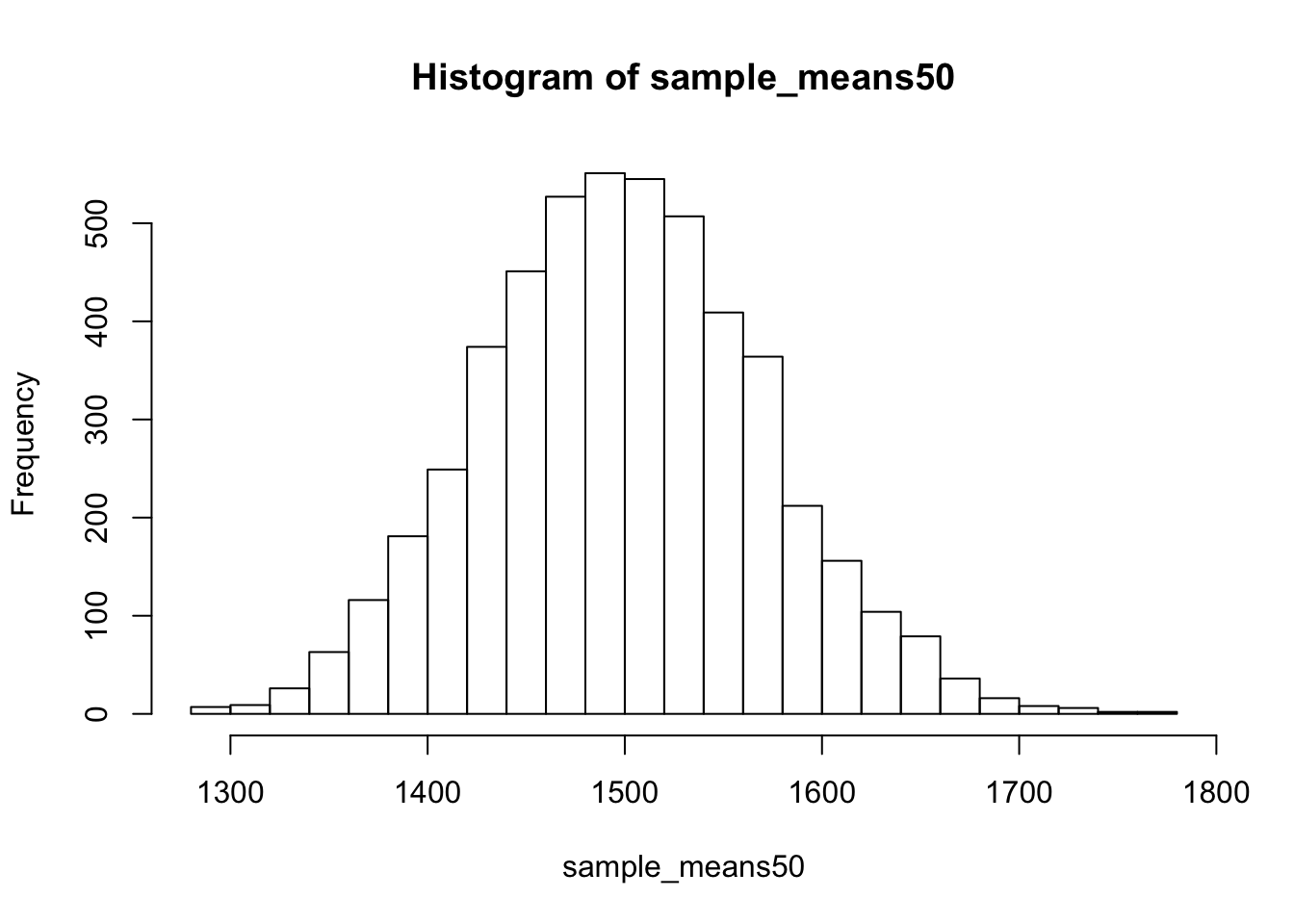#### November 9th, 2018

``````#library(DATA606)
#setwd("/Users/elinaazrilyan/Documents/Fall 2018/Data 606/Lab4/")
#startLab('Lab4a')``````

In this lab, we investigate the ways in which the statistics from a random sample of data can serve as point estimates for population parameters. We’re interested in formulating a sampling distribution of our estimate in order to learn about the properties of the estimate, such as its distribution.

## The data

We consider real estate data from the city of Ames, Iowa. The details of every real estate transaction in Ames is recorded by the City Assessor’s office. Our particular focus for this lab will be all residential home sales in Ames between 2006 and 2010. This collection represents our population of interest. In this lab we would like to learn about these home sales by taking smaller samples from the full population. Let’s load the data.

``load("more/ames.RData")``

We see that there are quite a few variables in the data set, enough to do a very in-depth analysis. For this lab, we’ll restrict our attention to just two of the variables: the above ground living area of the house in square feet (`Gr.Liv.Area`) and the sale price (`SalePrice`). To save some effort throughout the lab, create two variables with short names that represent these two variables.

``````area <- ames\$Gr.Liv.Area
price <- ames\$SalePrice``````

Let’s look at the distribution of area in our population of home sales by calculating a few summary statistics and making a histogram.

``summary(area)``
``````##    Min. 1st Qu.  Median    Mean 3rd Qu.    Max.
##     334    1126    1442    1500    1743    5642``````
``hist(area)``1. Describe this population distribution.

## The unknown sampling distribution

In this lab we have access to the entire population, but this is rarely the case in real life. Gathering information on an entire population is often extremely costly or impossible. Because of this, we often take a sample of the population and use that to understand the properties of the population.

If we were interested in estimating the mean living area in Ames based on a sample, we can use the following command to survey the population.

``samp1 <- sample(area, 50)``

This command collects a simple random sample of size 50 from the vector `area`, which is assigned to `samp1`. This is like going into the City Assessor’s database and pulling up the files on 50 random home sales. Working with these 50 files would be considerably simpler than working with all 2930 home sales.

1. Describe the distribution of this sample. How does it compare to the distribution of the population?
``summary(samp1)``
``````##    Min. 1st Qu.  Median    Mean 3rd Qu.    Max.
##     630    1161    1438    1516    1726    2868``````
``hist(samp1)``The distribution of the population is similar to that of our sample, it is not identical - but it is close. The mean and median are close enough.

If we’re interested in estimating the average living area in homes in Ames using the sample, our best single guess is the sample mean.

``mean(samp1)``
``##  1515.88``

Depending on which 50 homes you selected, your estimate could be a bit above or a bit below the true population mean of 1499.69 square feet. In general, though, the sample mean turns out to be a pretty good estimate of the average living area, and we were able to get it by sampling less than 3% of the population.

1. Take a second sample, also of size 50, and call it `samp2`. How does the mean of `samp2` compare with the mean of `samp1`? Suppose we took two more samples, one of size 100 and one of size 1000. Which would you think would provide a more accurate estimate of the population mean?
``````samp2 <- sample(area, 50)

#Let's calculate the mean of sample 2
mean(samp2)``````
``##  1470.62``

The mean of sample 2 happens to be closer to the true population mean. If we took a sample of size 100 and 1000 - we would expect the larger sample to provide a more accurate estimate of population mean. Let’s test this out.

``````samp3 <- sample(area, 100)

#Let's calculate the mean of sample 3
mean(samp3)``````
``##  1516.73``
``````samp4 <- sample(area,1000)

#Let's calculate the mean of sample 4
mean(samp4)``````
``##  1475.478``

My assumption is confirmed by the 1000 sample above - the mean is very close to true mean.

Not surprisingly, every time we take another random sample, we get a different sample mean. It’s useful to get a sense of just how much variability we should expect when estimating the population mean this way. The distribution of sample means, called the sampling distribution, can help us understand this variability. In this lab, because we have access to the population, we can build up the sampling distribution for the sample mean by repeating the above steps many times. Here we will generate 5000 samples and compute the sample mean of each.

``````sample_means50 <- rep(NA, 5000)

for(i in 1:5000){
samp <- sample(area, 50)
sample_means50[i] <- mean(samp)
}

hist(sample_means50)``````If you would like to adjust the bin width of your histogram to show a little more detail, you can do so by changing the `breaks` argument.

``hist(sample_means50, breaks = 25)``Here we use R to take 5000 samples of size 50 from the population, calculate the mean of each sample, and store each result in a vector called `sample_means50`. On the next page, we’ll review how this set of code works.

1. How many elements are there in `sample_means50`? Describe the sampling distribution, and be sure to specifically note its center. Would you expect the distribution to change if we instead collected 50,000 sample means?

There are 5000 elements in “sample_means50”. The distribution is Normal and the center is around 1500. The distribution will not change very much if we collected 50,000 sample means - 5000 is a large enough sample size for this exercise.

## Interlude: The `for` loop

Let’s take a break from the statistics for a moment to let that last block of code sink in. You have just run your first `for` loop, a cornerstone of computer programming. The idea behind the for loop is iteration: it allows you to execute code as many times as you want without having to type out every iteration. In the case above, we wanted to iterate the two lines of code inside the curly braces that take a random sample of size 50 from `area` then save the mean of that sample into the `sample_means50` vector. Without the `for` loop, this would be painful:

``````sample_means50 <- rep(NA, 5000)

samp <- sample(area, 50)
sample_means50 <- mean(samp)

samp <- sample(area, 50)
sample_means50 <- mean(samp)

samp <- sample(area, 50)
sample_means50 <- mean(samp)

samp <- sample(area, 50)
sample_means50 <- mean(samp)``````

and so on…

With the for loop, these thousands of lines of code are compressed into a handful of lines. We’ve added one extra line to the code below, which prints the variable `i` during each iteration of the `for` loop. Run this code.

``````sample_means50 <- rep(NA, 5000)

for(i in 1:5000){
samp <- sample(area, 50)
sample_means50[i] <- mean(samp)
#  print(i)
}``````

Let’s consider this code line by line to figure out what it does. In the first line we initialized a vector. In this case, we created a vector of 5000 zeros called `sample_means50`. This vector will will store values generated within the `for` loop.

The second line calls the `for` loop itself. The syntax can be loosely read as, “for every element `i` from 1 to 5000, run the following lines of code”. You can think of `i` as the counter that keeps track of which loop you’re on. Therefore, more precisely, the loop will run once when `i = 1`, then once when `i = 2`, and so on up to `i = 5000`.

The body of the `for` loop is the part inside the curly braces, and this set of code is run for each value of `i`. Here, on every loop, we take a random sample of size 50 from `area`, take its mean, and store it as the \(i\)th element of `sample_means50`.

In order to display that this is really happening, we asked R to print `i` at each iteration. This line of code is optional and is only used for displaying what’s going on while the `for` loop is running.

The `for` loop allows us to not just run the code 5000 times, but to neatly package the results, element by element, into the empty vector that we initialized at the outset.

1. To make sure you understand what you’ve done in this loop, try running a smaller version. Initialize a vector of 100 zeros called `sample_means_small`. Run a loop that takes a sample of size 50 from `area` and stores the sample mean in `sample_means_small`, but only iterate from 1 to 100. Print the output to your screen (type `sample_means_small` into the console and press enter). How many elements are there in this object called `sample_means_small`? What does each element represent?
``````sample_means_small <- rep(0, 100)

for(i in 1:100){
samp <- sample(area, 50)
sample_means_small[i] <- mean(samp)
}

#Let's take a look at our data

sample_means_small``````
``````##    1496.60 1405.26 1393.54 1613.16 1427.28 1568.36 1451.46 1598.96
##    1536.16 1552.20 1541.26 1475.48 1593.72 1512.30 1495.62 1523.10
##   1569.40 1489.98 1533.52 1464.02 1536.00 1454.10 1498.68 1534.66
##   1505.02 1646.64 1638.78 1437.06 1569.00 1507.46 1538.00 1398.42
##   1487.90 1599.24 1498.06 1488.50 1387.24 1397.70 1598.00 1514.24
##   1567.82 1531.20 1494.72 1503.44 1465.74 1446.70 1465.98 1511.64
##   1540.38 1642.30 1407.38 1413.22 1534.94 1587.30 1461.32 1584.38
##   1464.90 1486.90 1521.26 1535.26 1493.30 1439.44 1563.28 1407.64
##   1535.16 1609.86 1587.40 1371.72 1475.04 1458.52 1582.88 1468.34
##   1509.60 1415.58 1435.20 1446.78 1371.86 1480.54 1379.22 1399.80
##   1482.52 1445.30 1490.18 1522.94 1455.42 1354.04 1473.76 1464.88
##   1591.98 1575.48 1576.34 1417.18 1613.58 1411.08 1684.40 1549.74
##   1631.84 1537.22 1473.44 1477.26``````

There are a 100 elements in “sample_means_small” object. Each element is a mean of a random sample of 50 areas from our data.

## Sample size and the sampling distribution

Mechanics aside, let’s return to the reason we used a `for` loop: to compute a sampling distribution, specifically, this one.

``hist(sample_means50)``